# 5G与毫米波

$f = \frac{c}{\lambda}, \;\;c = 3 \times10^8m/s.$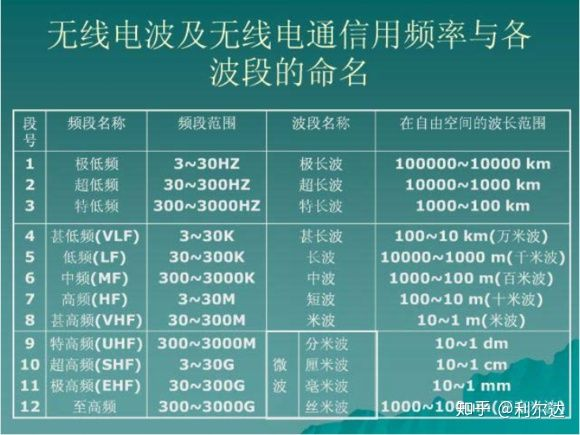# 毫米波与Massive MIMO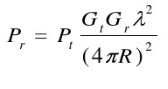(Gt， Gr, Pt, Pr分别代表发送，接受的天线增益，功率。）可以看到，接收功率与波长成正比。而毫米波更短的波长，也意味着更高的传输损耗（接收功率变小）。不止如此，毫米波的穿透等能力也大大降低，甚至连雨天的水滴都能对传输造成干扰。这一方面的传输损耗介绍在众多论文中比比皆是，这里不再给出详细介绍。我们只需要记得是，相比现在4G LTE的传输载波，毫米波的使用虽然带来了更广阔的带宽，却也饱受传输损耗之苦，如何解决在不大幅提升发送功率的前提下增大接收信噪比，就成了当务之急。

## MIMO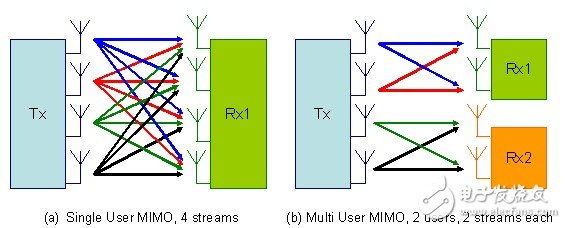MIMO对传输的增益个人认为非常容易理解。 两个人办公（$1\times1$的普通通信系统）的速度肯定是比不上四个人办公($2\times 2$的MIMO系统）的效率的。虽然这四个人可能由于配合原因（不同天线间数据流信息的相互干扰）有所损失，但稍加训练（发送预处理和接收均衡）想碾压两个人还是没有压力的。而正如我们一直熟知的那样，人多力量大，天线越多（比如$100\times 100$的大规模MIMO），增益也会越大。这就是Massive MIMO提出的初衷。

$L \ge 0.5\lambda$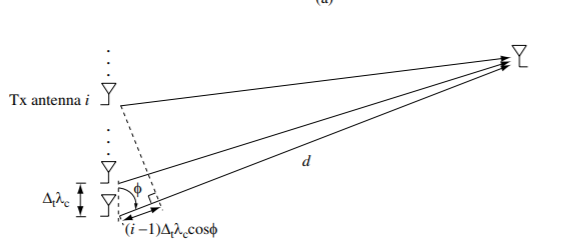## 波束赋形（波束成形， beamforming）

• 一般的天线发送信号，没有指定的角度，而是以一种比较无脑的方式，几乎是360度往空间各个方向发送信号。干扰了别人要接收的信号不说，哪怕从一个自私的角度出发，也浪费了大量的发送功率。就好像以前自习课你和同桌聊天，非要喊得全班都听见，既让自己消耗了更多的卡路里，也吵到其他人学习。

• 前面说到，MIMO的增益本质上就是人多力量大，然而人与人之间的配合不默契会导致一定的损耗，难以达到$1 + 1 =2$的效果。比如空间中其实有多条独立的路径可以到达接收端，明明大家可以各走一条，但如果挤在一起，反而会互相造成干扰。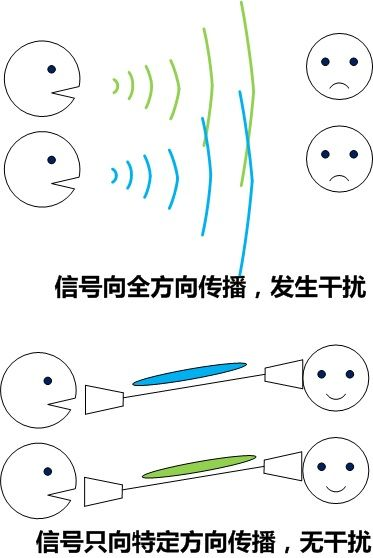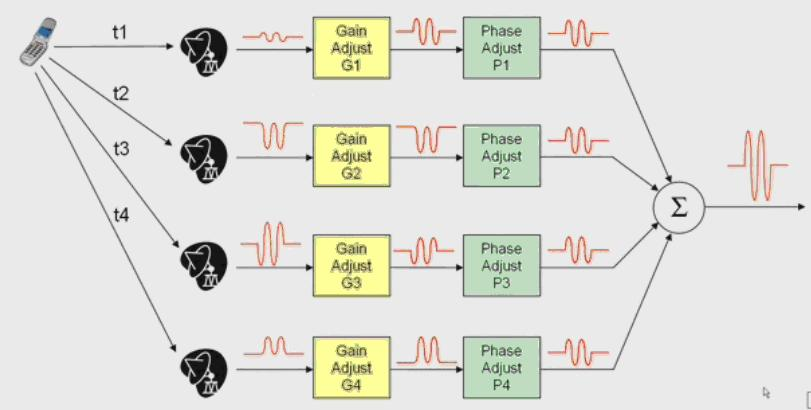## 波束成形的数学建模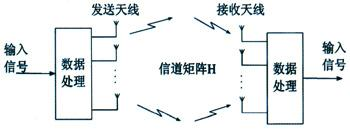$\mathbf{r} = \mathbf{W}^H\mathbf{H}\mathbf{V}\mathbf{s}.$

$\mathrm{min}\;\; E\{||\mathbf{W}^H\mathbf{H}\mathbf{V}\mathbf{s} - \mathbf{s}||^2\} \\ s.t. \;\;\;\;||\mathbf{V}||^2\le P$

# 5G： 混合波束赋形的机遇与挑战

**MIMO是5G前就提出的，波束成形也是。**那么5G的技术突破在哪里呢？

4G是从$1\times1$$2\times2$, 而5G是从$2\times2$$100\times100$. 虽然创新性也许没有前者那么颠覆性，但同样不可忽略。因为$2\times2$$100\times100$，并不是一个straightforward的过程。

©️2019 CSDN 皮肤主题: 大白 设计师: CSDN官方博客